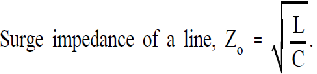• Thermal limitation (I2R limitation)
• Voltage regulation
• Stability Limitation

This is defined as the load (of unity power factor) that can be delivered by the line of negligible resistance.Where VLL is the receiving end voltage in kV and Zo is the surge impedance in ohms, and SIL is the surge impedance loading or natural loading of the line

The above  expression gives a limit of the maximum power that can be delivered by a line and is useful in designing the transmission line. This can be used for the comparison of loads that can be carried on the transmission lines at different voltages

From the above expression power transmitted through a long transmission lines can be either increased by increasing the value of the receiving end line voltage (VLL) or by reducing the surge impedance (Zo). Voltage transmission capability is increased day by day, this is the most commonly adopted method for increasing the power limit of the heavily loaded transmission line. But there is a limit beyond which is neither economical nor practical to increase the receiving end line voltage

By applying some methods such as introducing series capacitors (capacitors in series with the transmission line) or shunt capacitors (capacitors in parallel with transmission lines) can be used to reduce the value of surge impedance (Zo).Surge Impedance Loading (SIL) can be increased by reducing the Surge impedance of the line. From the above expression Zo can be decreased by either increasing the capacitance (C) of the line or by reducing the inductance (L) of the line. Inductance (L) of the transmission line cannot be reduced easily

By use of the series capacitors surge impedance (Zo) and the phase shift get reduced due to decrease in the line inductance (L). This improves the system stability limit. These capacitors also helps in reducing the line drops and so voltage variations. But this method causes difficulty under short circuit conditions of system as capacitors will get damage.

By use of shunt capacitors though the surge impedance (Zo) is reduced but the phase shift of the system increases this affects the poor stability in the system specially when synchronous machines are under the load. This method is not employed in long transmission lines specially when stability limits are present